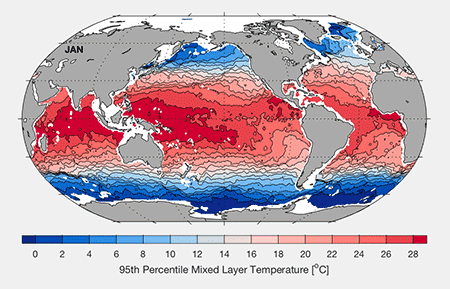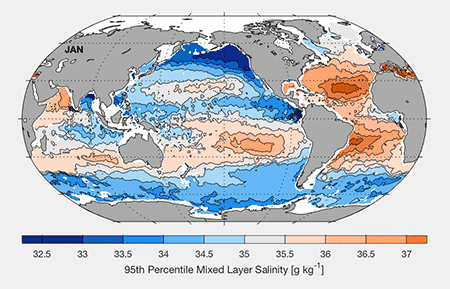# GOSML: A Global Ocean Surface Mixed Layer Statistical Monthly Climatology

GOSML is a global ocean surface mixed layer statistical monthly climatology of depth, temperature, and salinity that includes means; variances; values at depth-sorted 95th, 50th, and 5th percentiles; as well as skewness and kurtosis. Mixed layer property distributions are generally not normal, and the skewness and kurtosis provide assessments of the departure from normal distributions. To produce the percentile values, the local mixed layer values are sorted by depth, weighted by spatial, temporal, and bottom depth proximity, and then smoothed before selecting values at 95th, 50th, and 5th percentiles. The 95th percentile values are recommended as being representative of mixed layer properties ventilating the subsurface ocean, below the mixed layer.

## Seasonal Cycle Animations

[Click or tap on map graphics to animate]Monthly 95th Percentile DepthsMonthly Conservative Temperature for 95th Percentile DepthsMonthly Absolute Salinity for 95th Percentile Depths

This way to the GOSML map room

GOSML is described in the manuscript just below. Please click on the title for a link to the accepted version of this manuscript:

Johnson, G. C. and J. M. Lyman. 2022. GOSML: A Global Ocean Surface Mixed Layer Statistical Monthly Climatology: Means, Percentiles, Skewness, and Kurtosis. Journal of Geophysical Research, 127, e2021JC018219. https://doi.org/10.1029/2021JC018219.

Disclaimer:

Feel free to use GOSML for any non-commercial purposes, but do so at your own risk, we cannot take any liability on the use of GOSML.

Acknowledging use:

Please cite Johnson and Lyman (2022) when using GOSML.

Global monthly x 1° x 1° netCDF files with axes of decimal year (e.g., 0.5 = mid-January), longitude, and latitude and statistics for mixed layer depth, absolute salinity, conservative temperature, and potential density estimates for those axis locations. Individual files are provided below for each statistic: means, variances, skewness, kurtosis, 5th, 50th, and 95th percentiles, as well as a file for mapping statistic (number of profiles used, sum of weights, etc.). A Readme file is provided as well.

Variables in mixed_layer_properties_95.nc:

conservative_temperature_95== Conservative Temperature (°C)
absolute_salinity_95= Absolute Salinity (g/kg)
depth_95= Mixed Layer Depth (m)
potential_density_95 = Potential Density (kg/m^3)

Variables in mixed_layer_properties_50.nc:

conservative_temperature_50== Conservative Temperature (°C)
absolute_salinity_50= Absolute Salinity (g/kg)
depth_50= Mixed Layer Depth (m)
potential_density_50= Potential Density (kg/m^3)

Variables in mixed_layer_properties_5.nc:

conservative_temperature_5== Conservative Temperature (°C)
absolute_salinity_5= Absolute Salinity (g/kg)
depth_5= Mixed Layer Depth (m)
potential_density_5 = Potential Density (kg/m^3)

Variables in mixed_layer_properties_kurtosis.nc:

conservative_temperature_kurtosis = Conservative Temperature
absolute_salinity_kurtosis = Absolute Salinity
depth_kurtosis = Mixed Layer Depth
potential_density_kurtosis = Potential Density

Variables in mixed_layer_properties_skewness.nc:

conservative_temperature_skewness = Conservative Temperature
absolute_salinity_skewness = Absolute Salinity
depth_skewness = Mixed Layer Depth
potential_density_skewness = Potential Density

Variables in mixed_layer_properties_variance.nc:

conservative_temperature_variance= Conservative Temperature ((°C)^2)
absolute_salinity_variance = Absolute Salinity ((g/kg)^2)
depth_variance = Mixed Layer Depth (m^2)
potential_density_variance = Potential Density ((kg/m^3)^2)

Variables in mixed_layer_properties_mean.nc:

conservative_temperature_mean = Conservative Temperature (°C)
absolute_salinity_mean = Absolute Salinity (g/kg)
depth_mean = Mixed Layer Depth (m)
potential_density_mean = Potential Density (kg/m^3)

Variables in mixed_layer_mapping_stats.nc:

mapped_latitude = mapped (weighted) latitude
mapped_longitude = mapped (weighted) longitude
sum_mapping_weights = Summed Weights (effective) number of profiles
number_observations= Number of profiles